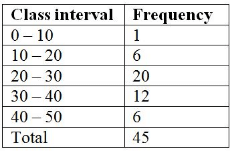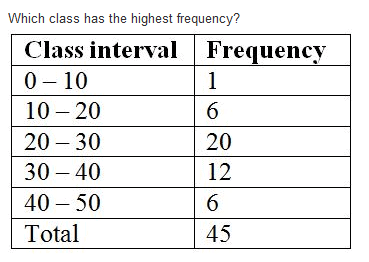Test: Data Handling- 1

# Test: Data Handling- 1

Test Description

## 10 Questions MCQ Test Mathematics (Maths) Class 8 | Test: Data Handling- 1

Test: Data Handling- 1 for Class 8 2022 is part of Mathematics (Maths) Class 8 preparation. The Test: Data Handling- 1 questions and answers have been prepared according to the Class 8 exam syllabus.The Test: Data Handling- 1 MCQs are made for Class 8 2022 Exam. Find important definitions, questions, notes, meanings, examples, exercises, MCQs and online tests for Test: Data Handling- 1 below.
Solutions of Test: Data Handling- 1 questions in English are available as part of our Mathematics (Maths) Class 8 for Class 8 & Test: Data Handling- 1 solutions in Hindi for Mathematics (Maths) Class 8 course. Download more important topics, notes, lectures and mock test series for Class 8 Exam by signing up for free. Attempt Test: Data Handling- 1 | 10 questions in 10 minutes | Mock test for Class 8 preparation | Free important questions MCQ to study Mathematics (Maths) Class 8 for Class 8 Exam | Download free PDF with solutions
 1 Crore+ students have signed up on EduRev. Have you?
Test: Data Handling- 1 - Question 1

### The number of times an observation occurs in a data is called its

Detailed Solution for Test: Data Handling- 1 - Question 1

The frequency  of a particular data value is the number of times the data value occurs. For example, if four students have a score of 80 in mathematics, and then the score of 80 is said to have a frequency of 4. The  frequency of a data value is often represented by ' f '.

Test: Data Handling- 1 - Question 2

### In the class- interval 70-80, 80 is the

Detailed Solution for Test: Data Handling- 1 - Question 2

Upper limit is the highest number of the interval. Hence 80 is the upper limit.

Test: Data Handling- 1 - Question 3

### The class mark of 95-100 is

Detailed Solution for Test: Data Handling- 1 - Question 3

Class mark is the midpoint of the upper and lower limits.
so for the given problem;

the class mark is
[ (95+100)÷2 ] = 97.5
Thus class mark is 97.5

Test: Data Handling- 1 - Question 4

The difference between the upper and lower limit is called

Detailed Solution for Test: Data Handling- 1 - Question 4

Class Size: Difference between the true upper limit and true lower limit of a class interval is called the class size.

Class size remains the same for all class intervals.

For the class interval 10 - 20

Class size is 10, i.e., (20 - 10 = 10)

Test: Data Handling- 1 - Question 5

The shape of a pie-chart is

Detailed Solution for Test: Data Handling- 1 - Question 5

A pie chart is circular in shape which uses pie slices to show relative sizes of data

Test: Data Handling- 1 - Question 6

The pie-chart is divided into

Detailed Solution for Test: Data Handling- 1 - Question 6

A pie chart is a circular chart divided into wedge-like sectors, illustrating proportion. Each wedge represents a proportionate part of the whole, and the total value of the pie is always 100 percent. Pie charts can make the size of portions easy to understand at a glance.

Test: Data Handling- 1 - Question 7

The central total angle in a pie chart is

Test: Data Handling- 1 - Question 8

18 out of 36 people love reading, so reading in the pie chart will be represented by

Detailed Solution for Test: Data Handling- 1 - Question 8

When we find the central angle on pie chart we get 18/36 * 360 =180 degree, which is half of complete angle 360. so people loveing reading can be represented by semi circular

Test: Data Handling- 1 - Question 9

What is the size of class intervals?Test: Data Handling- 1 - Question 10Detailed Solution for Test: Data Handling- 1 - Question 10

20 has the highest frequency as option b because as you have seen and frequency table the highest frequency is friendly only so it shows that it has the highest frequency and don't you feel bad I may tell you one thing this thing should not be asked you know these are very easy and you can see just a frequency table.

## Mathematics (Maths) Class 8

187 videos|323 docs|48 tests
 Use Code STAYHOME200 and get INR 200 additional OFF Use Coupon Code
Information about Test: Data Handling- 1 Page
In this test you can find the Exam questions for Test: Data Handling- 1 solved & explained in the simplest way possible. Besides giving Questions and answers for Test: Data Handling- 1, EduRev gives you an ample number of Online tests for practice

## Mathematics (Maths) Class 8

187 videos|323 docs|48 tests